Courses

Simple Harmonic Motion NAT Level - 2

10 Questions MCQ Test Topic wise Tests for IIT JAM Physics | Simple Harmonic Motion NAT Level - 2

Description
Attempt Simple Harmonic Motion NAT Level - 2 | 10 questions in 45 minutes | Mock test for IIT JAM preparation | Free important questions MCQ to study Topic wise Tests for IIT JAM Physics for IIT JAM Exam | Download free PDF with solutions
*Answer can only contain numeric values
QUESTION: 1

A particle of mass m moves in one dimensional potential v(x)=-ax2 + bx4 where a and b are positive constants. What is the angular frequency of small oscillations about the minimum of the potential in units of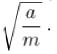Solution: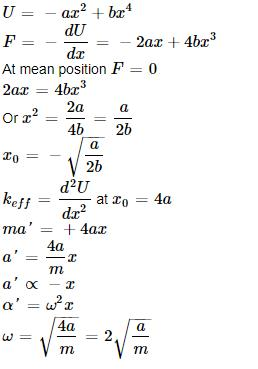*Answer can only contain numeric values
QUESTION: 2

A spring of force constant k is stretched a certain distance. It takes twice as much work to strech a second spring by half this distance. What is the force constant of the second spring? (in units of k)

Solution: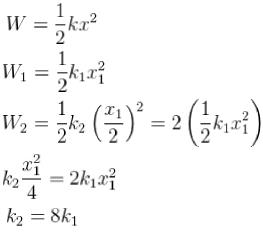*Answer can only contain numeric values
QUESTION: 3

Figure shows block 1 of mass 0.2 g sliding to the right over a frictionless elevated surface at a speed of 8 m/s. The block undergoes an elastic collision with stationary block 2, which is attached to a spring of constant 1200 N/m. After the collision, block 2 oscillates in SHM with a period of 0.2 s & block 1 slides off the opposite end of the elevated surface, surface falling height = 6m find the value of d in meters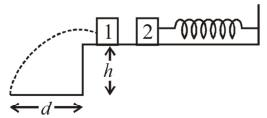Solution:

Time period of a SHM,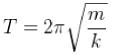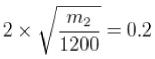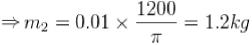Now, we can find the rebound speed of the block 1 after collision,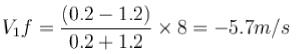Time of flight =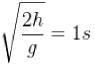d = velocity x time
= 5.7 x 1 = 5.7 m

*Answer can only contain numeric values
QUESTION: 4

What is the period of oscillation of a physical pendulum which consists of a circular hoop hanging from a nail an a wall the mass of the hoop is 3 kg and its radius is 20 cm, if it displaced slightly by a passing breeze? (in seconds)

Solution:

Parallel axis theorem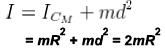d → distance from pivot to centre of mass.
Period of physical pendulum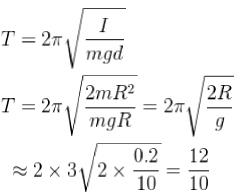= 1.2 seconds

*Answer can only contain numeric values
QUESTION: 5

A long straight and massless rod pivots about one end in a vertical plane. In configuration I, two small identical masses are attached to the free end, in the second configuration one mass is attached at the centre and the other is attached to the free end. What is the ratio of frequency of small oscillations of configuration / to that of configuration II?

Solution: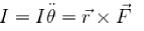I = moment of inertia
r = moment arm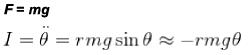(for small angles)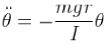∴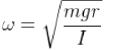For the first system
l1 = 2mr2
radius of gyration = r1, = 2r
For the second system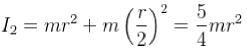radius of gyration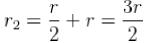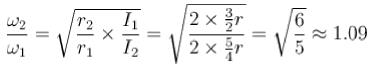*Answer can only contain numeric values
QUESTION: 6

Two identical springs with spring constant k are connected to identical masses of mass in, as shown in the figures below the ratio of the period for the springs connected in parallel (figure I) to the period for the springs connected in series (figure 2) is what?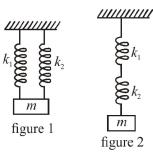Solution:

Parallel
Effective spring constant = k1+ k2 = kp
Series
Effective spring constant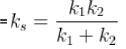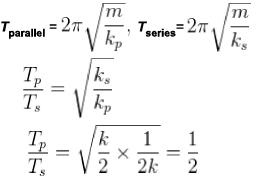*Answer can only contain numeric values
QUESTION: 7

What is the phase constant in degrees for the harmonic oscillator with the velocity function V(f) given in figure if the position function x(t) has the form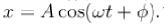The vertical axis scale is set by Vs = 4 cm /s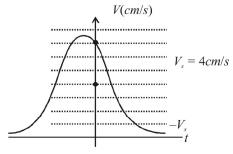Solution: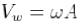where → amplitude of oscillator
Vm = 5 cm/s since Vs= 4 cm/s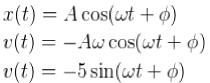from the graph, at t = 0, v = 4 cm/s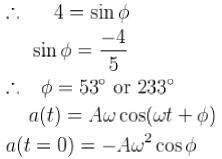If we take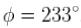,acceleration would be positive but from the graph since the v is decreasing at
t = 0
∴ the acceleration should be negative!
∴ Ø = 53°

*Answer can only contain numeric values
QUESTION: 8

For a simple pendulum, find the angular displacement at which the restoring torque required for simple harmonic motion deviates from the actual restoring torque by 2% use g = 10m/s2 . (θ in degrees).

Solution:

The restoring torque on the bob of the pendulum at an angle with the vertical is given by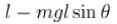We apply small angle approximation to so that the motion can be followed as simple harmonic motion, the torque becomes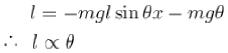The problem requires to find the value of 9 for which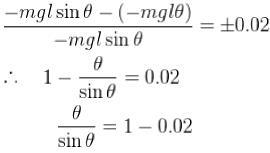Write the taylor expansion of Sin θ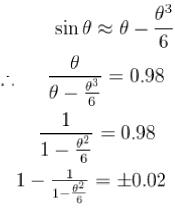Taking absolute value on both sides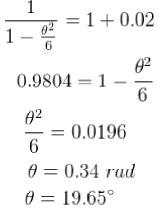*Answer can only contain numeric values
QUESTION: 9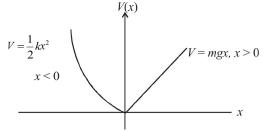A particle of mass m moves in the potential shown above find the period of the motion when the particle has energy E. (in terms of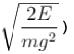Solution:

For the simple harmonic oscillator (SHO) part,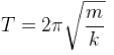Since the graph shows only half of the usual SHO potential, the period contribution from the SHO part should be half the usual period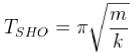For the gravitational potential, one can calculate the period from the usual kinematics equation for constant acceleration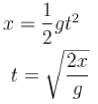Since E is always conserved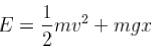At the end point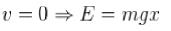x = Elm g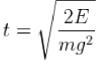Since the particle has to travel from the origin to the right end point & then back again the total time contribution is twice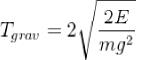*Answer can only contain numeric values
QUESTION: 10

The circular hoops, X & Y are hanging on nails in a wall. The mass of X is four times of Yand the diameter of X is also four times that of Y and the diameter of X is also four times that of Y. If the period of small oscillations of Xis T, then find the period of oscillations for Y (in terms T)

Solution:

Assuming both the loops to be point masses concentrated at the centre of mass, they can be considered as simple pendulums. For small oscillations, time period of a
simple pendulum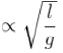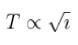Time period of Y is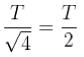Use Code STAYHOME200 and get INR 200 additional OFF Use Coupon Code

Track your progress, build streaks, highlight & save important lessons and more!

Similar ContentRelated tests